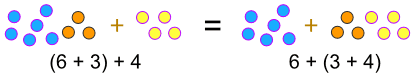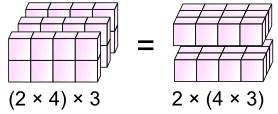Definition of

# Associative LawWhen adding it doesn't matter how we group the numbers (i.e. which we calculate first).

Example addition: (6 + 3) + 4 = 6 + (3 + 4)
Because 9 + 4 = 6 + 7 = 13

Also when multiplying it doesn't matter how we group the numbers.

Example multiplication: (2 × 4) × 3 = 2 × (4 × 3)
Because 8 × 3 = 2 × 12 = 24